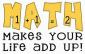# solve pls: number of club members for maximum revenueA student club on a college charges monthly membership dues of P10, less 5 centavos for each member over 60. How many members would give the club, the most revenue from the monthly dues?### Let

Let
R = monthly revenue
x = number of student-member in excess of 60
x + 60 = total number of student-member

For number of members ≤ 60
monthly dues for each member = P10

For number of members > 60
monthly dues for each member = P(10 - 0.05x)

$R = (10 - 0.05x)(60 + x)$

$R = 600 + 7x - 0.05x^2$

$\dfrac{dR}{dx} = 7 - 0.1x = 0$

$x = 70$

total number of members = 70 + 60 = 130       answer### thank you kaayu sir.

thank you kaayu sir.### way sapayan

way sapayan

• Mathematics inside the configured delimiters is rendered by MathJax. The default math delimiters are $$...$$ and $...$ for displayed mathematics, and $...$ and $...$ for in-line mathematics.